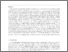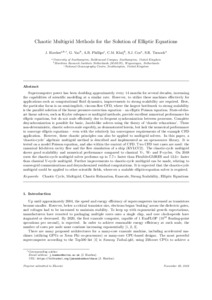nerc.ac.uk

# Chaotic multigrid methods for the solution of elliptic equations

Hawkes, J.; Vaz, G.; Phillips, A.B.; Klaij, C.M.; Cox, S.J.; Turnock, S.R.. 2018 Chaotic multigrid methods for the solution of elliptic equations. Computer Physics Communications, 237. 26-36. https://doi.org/10.1016/j.cpc.2018.10.031Preview
Text
ChaoticMultigridHawkes.pdf

## Abstract/Summary

Supercomputer power has been doubling approximately every 14 months for several decades, increasing the capabilities of scientific modelling at a similar rate. However, to utilize these machines effectively for applications such as computational fluid dynamics, improvements to strong scalability are required. Here, the particular focus is on semi-implicit, viscous-flow CFD, where the largest bottleneck to strong scalability is the parallel solution of the linear pressure-correction equation — an elliptic Poisson equation. State-of-the-art linear solvers, such as Krylov subspace or multigrid methods, provide excellent numerical performance for elliptic equations, but do not scale efficiently due to frequent synchronization between processes. Complete desynchronization is possible for basic, Jacobi-like solvers using the theory of ‘chaotic relaxations’. These non-deterministic, chaotic solvers scale superbly, as demonstrated herein, but lack the numerical performance to converge elliptic equations — even with the relatively lax convergence requirements of the example CFD application. However, these chaotic principles can also be applied to multigrid solvers. In this paper, a ‘chaotic-cycle’ algebraic multigrid method is described and implemented as an open-source library. It is tested on a model Poisson equation, and also within the context of CFD. Two CFD test cases are used: the canonical lid-driven cavity flow and the flow simulation of a ship (KVLCC2). The chaotic-cycle multigrid shows good scalability and numerical performance compared to classical V-, W- and F-cycles. On 2048 cores the chaotic-cycle multigrid solver performs up to faster than Flexible-GMRES and faster than classical V-cycle multigrid. Further improvements to chaotic-cycle multigrid can be made, relating to coarse-grid communications and desynchronized residual computations. It is expected that the chaotic-cycle multigrid could be applied to other scientific fields, wherever a scalable elliptic-equation solver is required.

Item Type: Publication - Article https://doi.org/10.1016/j.cpc.2018.10.031 00104655 15 Jan 2019 13:48 +0 (UTC) http://nora.nerc.ac.uk/id/eprint/522032View Item